• 基于MATLAB的IIR和FIR滤波器设计-实验4 FIR数字滤波器设计.doc ...（3）给出FIR数字滤波器的冲激响应，绘出它们的幅度相位频响曲线，讨论它们各自的实现形式特点。 例1 用凯塞窗设计一FIR低通滤波器，matlab
• 1、对目标频响进行平滑，在所需的频点上进行插值，可以使用cubic spline找到所需要的频点的gain； 2、目标频响可以是实数（零相位），也可以叠加任意的相位，如线性相位或者minimal phase； 3、对经过采样的目标...

比如，我们要设计一个有用户界面，有多个滑块的图示均衡器（graphic equalizer），滑块的位置表示每一个band的增益，将这些band的增益连接起来就是图示均衡器的幅频响应。可以使用如下的窗函数法：

1、对目标频响进行平滑，在所需的频点上进行插值，可以使用cubic spline找到所需要的频点的gain；

2、目标频响可以是实数（零相位），也可以叠加任意的相位，如线性相位或者minimal phase；

3、对经过采样的目标频响进行IFFT，获取目标脉冲响应；

4、设计一个窗函数，保证目标脉冲响应的主要的能量集中在窗函数里面；

6、比目标频率响应和第5步设计的加时域窗的频响的差异是否满足规格要求。

展开全文算法
• 基于MATLAB的IIR和FIR滤波器设计-实验5 基于MATLAB的数字滤波器设计.doc ...（3）给出FIR数字滤波器的冲激响应，绘出它们的幅度相位频响曲线，讨论它们各自的实现形式特点。 例1 用凯塞窗设计一FIR低通滤波器，matlab
• b=1;a=[843,-654519,-588988,-523459,-457934,-392411,-326891,-195838,-64787,66262,328381,590498,918149,1311335,1835592,2359848,3080711,3801575,4719046,5702053,6750596,7930212,9240901,10682663,12189962,1...

b=1;

a=[843,-654519,-588988,-523459,-457934,-392411,-326891,-195838,-64787,66262,328381,590498,918149,1311335,1835592,2359848,3080711,3801575,4719046,5702053,6750596,7930212,9240901,10682663,12189962,13828334,15597779,17432762,19333283,21299341,23330937,25428071,27590743,29753416,31916090,34013231,36175908,38273052,40304660,42270734,44171273,45940741,47579137,49151999,50528253,51773435,52822010,53673977,54394872,54853623,55181302]

fvtool(b,a);

单击工具栏第三个按钮,就可以观察到幅频特性,

向左转|向右转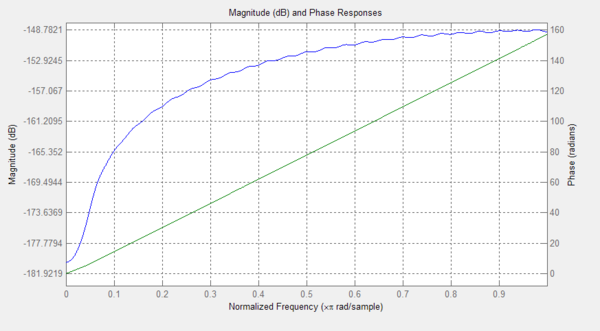展开全文一，写在前面的话.

学习了以下链接，然后写出了MATLAB代码，在本文末，大家可以随便下载代码，进行尝试，我代码调试正常，可使用。

1. 如果你是初学者，建议你看一下这个链接https://blog.csdn.net/zhoufan900428/article/details/8969470

自学什么是窗函数，什么是滤波器，为啥要用窗函数，怎么计算一些值，怎么根据这些值选择窗函数。

2. 如果你明白了上述内容，但还不能单独写代码进行分析，推荐你看这个链接：https://www.cnblogs.com/xhslovecx/p/10196851.html

自学怎么计算，然后选好窗函数，就设计，写公式。在那文章末尾他给了MATLAB采用FIR I型设计20Hz以下的低通滤波器的代码。

3. 如果你明白了以上内容，那么就请看下去吧：

代码都上传到下面了，随便用，但如果你要发表的话，请改一改，因为我的论文已经入库了，你要是直接抄，老师可能从查重率上把你毙了，改个参数名啥的可以，写论文很少发代码的

二，简单介绍

我们知道IIR（无限脉冲响应）数字滤波器的设计方法是利用模拟滤波器成熟的理论及设计图表进行的，因而保留了一些典型模拟滤波器优良的幅度特性，但设计中只考虑到了幅度特性，没考虑到相位特性，所设计的滤波器相位特性一般是非线性的。而FIR（有限脉冲响应）滤波器在保证幅度特性满足技术要求的同时，比较容易做到有严格的线性相位特性。

FIR滤波器分为四种：LP(低通滤波器)，HP(高通滤波器)，BP(带通滤波器)，BS(带阻滤波器)。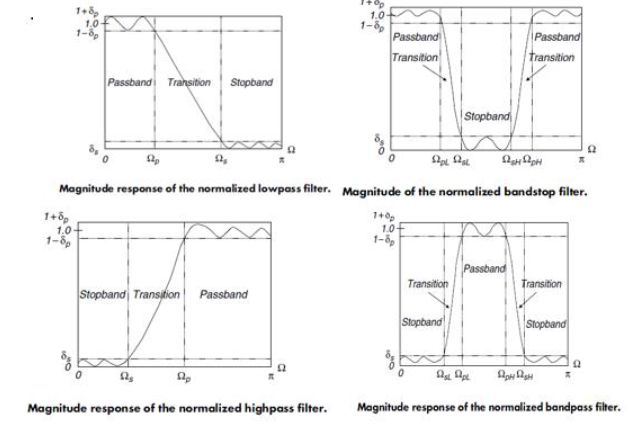它们的公式我就不写了，但是需要知道。还要知道什么是线性相位FIR滤波器幅度特性和零点分布特点。

我们熟知的窗函数有：三角窗，矩形窗，汉明窗，汉宁窗，布莱克曼窗，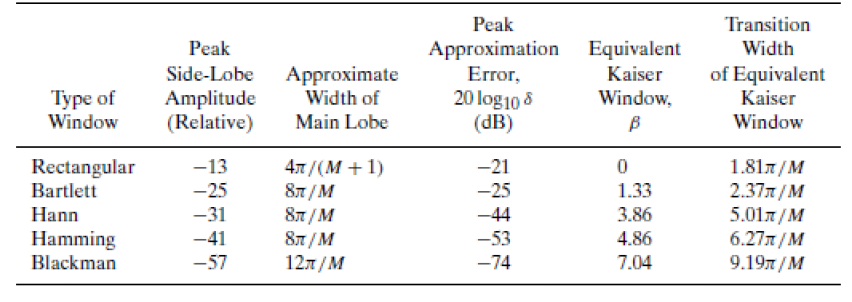我们若是分析特性，则需要知道什么是主瓣，旁瓣，若是选择窗函数，则需要计算窗长度N，然后选择合适的阻带衰减（dB）,阻带衰减不能比窗长度小。

然后写代码的时候，要手写时域表达式（这个叫写窗）。我把它们的公式写在下面：大家可以直接复制粘贴。

我直接贴了四个窗函数MATLAB的代码，请大家先参悟一下，再看加上滤波器的代码。

第一个是矩形窗：

n=0:1:14;
wR=ones(1,15);% 编写矩形窗
hd=sin(0.25*pi*(n-7+eps))./(pi*(n-7+eps));%读入hd（n）函数
h1=hd.*wR;%计算h（n）
N=64;
H1=fft(h1,N);%调用子程序计算H（k）
n=0:N-1;w=2*pi/64*n;subplot(2,2,1);
plot(w,fftshift(20*log10((abs(H1)))));%画幅度曲线
grid on
ylabel('20lg|H(jw)|/dB')
title('幅度曲线和相频曲线（n=15）');
n=0:N-1;w=2*pi/64*n;subplot(2,2,3);
plot(w,fftshift(unwrap(phase(H1))));%画相频曲线
grid
clear all;
n=0:1:32;
wR=ones(1,33);% 编写矩形窗
hd=sin(0.25*pi*(n-16+eps))./(pi*(n-16+eps));%读入hd（n）函数
h1=hd.*wR;%计算h（n）
N=64;
H1=fft(h1,N);%调用子程序计算H（k）
n=0:N-1;w=2*pi/64*n;subplot(2,2,2);
plot(w,fftshift(20*log10((abs(H1)))));%画幅度曲线
grid on
ylabel('20lg|H(jw)|/dB')
title('幅度曲线和相频曲线（n=15）');
n=0:N-1;w=2*pi/64*n;subplot(2,2,4);
plot(w,fftshift(unwrap(phase(H1))));%画相频曲线
grid

第二个是汉宁窗

clear all;
n=0:1:14;
wH=0.5*(1-cos(2*pi/14*n));% 编写汉宁窗
hd=sin(0.25*pi*(n-7+eps))./(pi*(n-7+eps));%读入hd（n）函数
h1=hd.*wH;%计算h（n）
N=64;
H1=fft(h1,N);%调用子程序计算H（k）
n=0:N-1;w=2*pi/64*n;subplot(2,2,1);
subplot(2,2,1);
plot(w,fftshift(20*log10((abs(H1)))));%画幅度曲线
grid
ylabel('20lg|H(jw)|/dB');
title('幅度曲线和相频曲线（n=15）');
n=0:N-1;w=2*pi/64*n;subplot(2,2,1);
subplot(2,2,3);
plot(w,fftshift(unwrap(phase(H1))));%画相频曲线
grid
n=0:1:32;
wH=0.5*(1-cos(2*pi/32*n));% 编写汉宁窗
hd=sin(0.25*pi*(n-16+eps))./(pi*(n-16+eps));%读入hd（n）函数
h1=hd.*wH;%计算h（n）
N=64;
H1=fft(h1,N);%调用子程序计算H（k）
n=0:N-1;w=2*pi/64*n;subplot(2,2,1);
subplot(2,2,2);
plot(w,fftshift(20*log10((abs(H1)))));%画幅度曲线
grid
ylabel('20lg|H(jw)|/dB')
title('幅度曲线和相频曲线（n=33）');
n=0:N-1;w=2*pi/64*n;subplot(2,2,1);
subplot(2,2,4);plot(w,fftshift(unwrap(phase(H1))));%画相频曲线
grid

第三个是汉明窗

clear all;
n=0:1:14;
wH=0.54-0.46*cos(2*pi*n/(14+eps));% 编写海明窗
hd=sin(0.25*pi*(n-7+eps))./(pi*(n-7+eps));%读入hd（n）函数
h1=hd.*wH;%计算h（n）
N=64;
H1=fft(h1,N);%调用子程序计算H（k）
n=0:N-1;w=2*pi/64*n;subplot(2,2,1);
subplot(2,2,1);
plot(w,fftshift(20*log10((abs(H1)))));%画幅度曲线
grid
ylabel('20lg|H(jw)|/dB');
title('幅度曲线和相频曲线（n=15）');
n=0:N-1;w=2*pi/64*n;subplot(2,2,1);
subplot(2,2,3);
plot(w,fftshift(unwrap(phase(H1))));%画相频曲线
grid
n=0:1:32;
wH=0.5*(1-cos(2*pi/32*n));% 编写汉宁窗
hd=sin(0.25*pi*(n-16+eps))./(pi*(n-16+eps));%读入hd（n）函数
h1=hd.*wH;%计算h（n）
N=64;
H1=fft(h1,N);%调用子程序计算H（k）
n=0:N-1;w=2*pi/64*n;subplot(2,2,1);
subplot(2,2,2);
plot(w,fftshift(20*log10((abs(H1)))));%画幅度曲线
grid
ylabel('20lg|H(jw)|/dB')
title('幅度曲线和相频曲线（n=33）');
n=0:N-1;w=2*pi/64*n;subplot(2,2,1);
subplot(2,2,4);plot(w,fftshift(unwrap(phase(H1))));%画相频曲线
grid

第四个是布莱克曼窗

n=0:14
wB=0.42-0.5*cos(2*pi/(14+eps)*n)+0.08*cos(4*pi/(14+eps)*n)
hd=sin(0.25*pi*(n-7+eps))./(pi*(n-7+eps))
h1=hd.*wB
H1=fft(h1,64)
n=0:63
w=2*pi*n/64
subplot(2,2,1)
plot(w,fftshift(20*log10((abs(H1)))))
grid
ylabel('20lg|H(jw)|/dB')
title('N=15幅度曲线')
subplot(2,2,3)
plot(w,unwrap(fftshift(phase(H1))))
grid
title('N=15相频曲线')
clear all
n=0:32
wB=0.42-0.5*cos(2*pi/(32+eps)*n)+0.08*cos(4*pi/(32+eps)*n)
hd=sin(0.25*pi*(n-16+eps))./(pi*(n-16+eps))
h1=hd.*wB
H1=fft(h1,64)
n=0:63
w=2*pi*n/64
subplot(2,2,2)
plot(w,fftshift(20*log10((abs(H1)))))
grid
ylabel('20lg|H(jw)|/dB')
title('N=33幅度曲线')
subplot(2,2,4)
plot(w,unwrap(fftshift(phase(H1))))
grid
title('N=33相频曲线')

希望大家结合公式与窗函数特性加上代码，参悟一下。

好了现在我们开始设计FIR滤波器，至于书面的计算过程我就不写了，又不懂得请参考本文“写在前面的话”的第一个链接的内容。

第一个例子，用汉明窗设计一个低通滤波器，Wp=0.2π, Ws=0.4π, Ap=0.25dB, As=50dB

%%%%%Write by Han%%%%%这是实时脚本文件%%%%%
close all;
clc
Wp=0.2*pi;
Ws=0.4*pi;
tr_width=Ws-Wp;                         %Transition zone width
N=ceil(6.6*pi/tr_width)+1               %Filter length
n=0:1:N-1;
Wc=(Ws+Wp)/2;                           %Cutoff frequency of ideal low-pass filter
hd = ideal_lp(Wc,N);                      %Unit impulse response of an ideal low-pass filter
w_ham=0.54-0.46*cos(2*pi*n/(14+eps));    % Writing a haming window
h=hd.*w_ham;                             %Truncated to the actual unit impulse response
[db,mag,pha,w]=freqz_m4(h,);          %Calculate the amplitude response of the actual filter
delta_w=2*pi/1000;
Ap=-(min(db(1:1:Wp/delta_w+1)))          %Actual passband ripple
As=-round(max(db(Ws/delta_w+1:1:501)))   %Actual stop band ripple
subplot(221)
stem(n,hd)                               %Matchstick figure
title('Ideal unit impulse response: hd(n)')
subplot(222)
stem(n,w_ham)
title('Hamming window: w(n)')
subplot(223)
stem(n,h)
title('Actual unit impulse response: h(n)')
subplot(224)
plot(w/pi,db)
title('Amplitude response: (dB)')
axis([0,1,-100,10])
%2 Custom functions used by this program
% One is the "freqz_m4.m"
% Another is the "ideal_lp.m"

我在这用了两个自定义函数，所以大家还需要新建两个.m文件，一个是“freqz_m4.m”文件名与函数名需要保持一致。

function[db,mag,pha,w]=freqz_m4(b,a)
[H,w]=freqz(b,a,1000,'whole');
H=(H(1:1:501));
w=(w(1:1:501));
mag=abs(H);
db=20*log10((mag+eps)/max(mag));
pha=angle(H);
end

另一个是“ideal_lp.m”

function hd=ideal_lp(Wc,N)
alpha= (N-1)/2;
n=0:1:N-1;
m=n-alpha+eps;
hd=sin (Wc*m)./(pi*m);
end

注意要把这三个文件保存在一个英文命名的文件夹中，不然MATLAB可能会出现.m文件打不开，或者找不到路径的问题。这个问题可参考（https://blog.csdn.net/Smile_h_ahaha/article/details/92477206）进行修正。代码是没问题的。

然后生成了以下图像：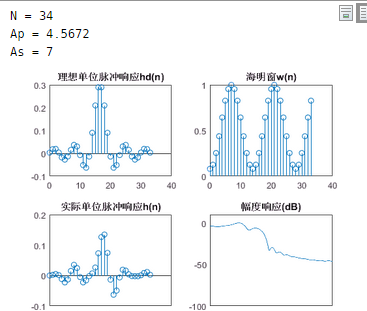第二个，我们设计个使用汉宁窗的高通滤波器，设计参数是：Wp=0.6 π,Ws=0.4 π,,Ap=0.25dB,As=40dB

%%%%%%%%%%%%%%% Write by Han %%%%%%%%%这是实时脚本文件%%%%%%%%%%%%
Wp=0.6*pi; Ws=0.4*pi;
tr_width=Wp-Ws;                         %Transition zone width
N=ceil (6.2*pi/tr_width) +1             %Filter length
n=0:1:N-1;
Wc= (Ws+Wp) /2;                         %The cut-off frequency of the ideal high-pass filter
hd=ideal_hp2(Wc,N);                      %Unit impulse response of an ideal high-pass filter
w_han=0.5*(1-cos(2*pi/14*n));            % Writing Hanning window
h=hd.*w_han;                            %Truncated to the actual unit impulse response
[db,mag,pha,w]=freqz_m4 (h,);         %Calculate the amplitude response of the actual filter
delta_w=2*pi/1000;
Ap=-(min(db(Wp/delta_w+1:1:500)))        %Actual passband ripple
As=-round(max(db(1:1:Wp/delta_w+1)))     %Actual stop band ripple
subplot(221)
stem(n,hd)                               %Matchstick figure
title('Ideal unit impulse response: hd(n)')
subplot(222)
stem(n,w_han)
title('Hanning: w(n)')
subplot(223)
stem(n,h)
title('Actual unit impulse response: h(n)')
subplot(224)
plot(w/pi,db)
title('FIR digital high-pass filter amplitude response: (dB)')
axis([0,1,-100,10])

这也用到两个自定义函数，

%%%%%%%%%%%%% ideal_hp2.m %%%%%%%%%%%%%
function hd=ideal_hp2(Wc,N)
alpha= (N-1)/2;
n=0:1:N-1;
m=n-alpha+eps;
hd=(sin (pi*m)-sin(Wc*m))./(pi*m);
%%%%%%%%%%%请再新建一个.m文件放以下部分%%%%%
function[db,mag,pha,w]=freqz_m4(b,a)
[H,w]=freqz(b,a,1000,'whole');
H=(H(1:1:501));
w=(w(1:1:501));
mag=abs(H);
db=20*log10((mag+eps)/max(mag));
pha=angle(H);
end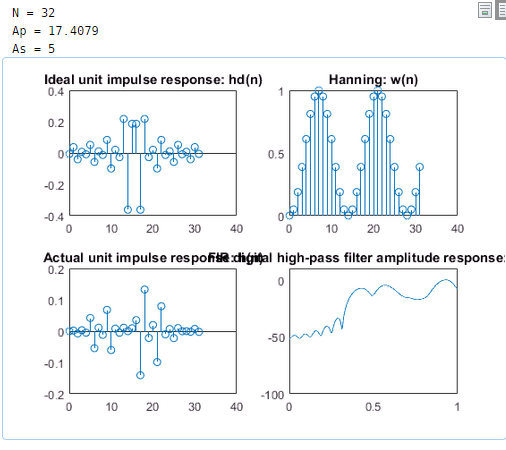第三个，我们用汉宁窗设计一个bandstop(带阻) FIR滤波器，设计参数是：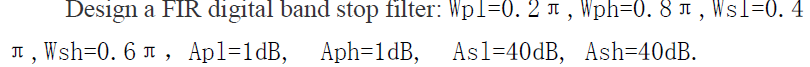%%%%%%%%%%%%%%%%%% Write by Han %%%%%%%%%%这是实时脚本文件%%%%%%%%%%%%
Wpl=0.2*pi; Wph=0.8*pi; Wsl=0.4*pi; Wsh=0.6*pi;
tr_width=min((Wsl-Wpl),(Wph-Wsh));
N=ceil (6.2*pi/tr_width)
n=0:1:N-1;
Wcl= (Wsl+Wpl) /2;                      %Lower cutoff frequency of ideal band stop filter
Wch= (Wsh+Wph) /2;                      %Upper cutoff frequency of ideal band stop filter
hd=ideal_bs(Wcl,Wch,N);                 %Unit impulse response of an ideal band stop filter
w_hann= 0.5*(1-cos(2*pi/14*n));                  %Hanning window
h=hd.*w_hann;                          %Truncated to the actual unit impulse response
[db,mag,pha,w]=freqz_m4 (h,);        %Calculate the amplitude response of the actual filter
delta_w=2*pi/1000;
Ap=-(min(db(1:1:Wpl/delta_w+1)))          %Actual passband ripple
As=-round(max(db(Wsl/delta_w+1:1:Wsh/delta_w+1)))      %Actual stop band ripple
subplot(221)
stem(n,hd)                                %Matchstick figure
title('Ideal: hd(n)')
subplot(222)
stem(n,w_hann)
title('hanning w(n)')
subplot(223)
stem(n,h)
title('Real h(n)')
subplot(224)
plot(w/pi,db)
title('FIRHanning window digital band rejection filter amplitude response(dB)')
axis([0,1,-100,10])
%%%%%%%%%%%%%%%%%%%%% ideal_bs.m %%%%%%%%新建一个.m文件%%%%%%%%%%%%

function hd=ideal_bs(Wcl,Wch,N)
alpha= (N-1) /2; n=0:1:N-1;
m=n-alpha+eps;
hd=(sin (Wcl*m) + sin(pi*m)-sin(Wch*m) ) ./(pi*m);
end
%%%%%%%%%%%%%%%%%%%%freqz_m4.m%%%%%%新建一个.m文件%%%%%%%%%%%
function[db,mag,pha,w]=freqz_m4(b,a)
[H,w]=freqz(b,a,1000,'whole');
H=(H(1:1:501));
w=(w(1:1:501));
mag=abs(H);
db=20*log10((mag+eps)/max(mag));
pha=angle(H);
end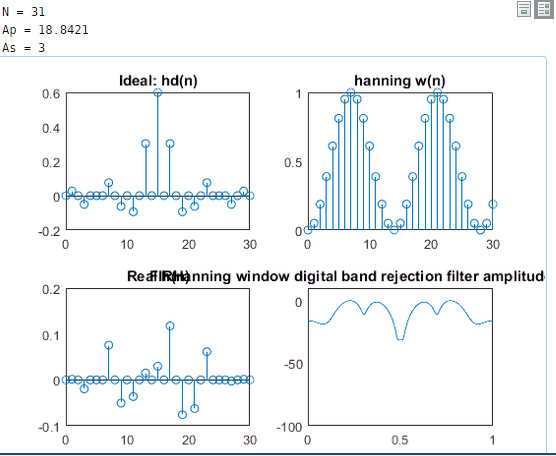其他的大家能参悟到这，其他的估计也就触类旁通了吧，学海无涯，兴趣作舟，祝大家好运！

展开全文MATLAB FIR滤波器设计 窗函数
• FIR数字滤波器设计（下）

千次阅读 2020-06-27 11:23:23
今天带来第三篇，FIR数字滤波器设计，包括窗函数法设计FIR滤波器、频率采样法设计FIR滤波器以及基于firls函数remez函数的最优化方法设计FIR滤波器。话不多说，上货。 这里也超链接了上、中两篇，方便参考学习。 ...

今天给大侠带来FIR数字滤波器设计，由于篇幅较长，分三篇。今天带来第三篇，FIR数字滤波器设计，包括窗函数法设计FIR滤波器、频率采样法设计FIR滤波器以及基于firls函数和remez函数的最优化方法设计FIR滤波器。话不多说，上货。

这里也超链接了上、中两篇，方便参考学习。

FIR数字滤波器设计（上）

FIR数字滤波器设计（中）数字滤波器的输入输出均为数字信号，信号通过数字滤波器后，可以改变频率成分的相对比例或滤除某些频率成分。数字滤波器可以分为IIR数字滤波器和FIR数字滤波器。

本篇只介绍FIR数字滤波器的设计，可以根据所给定的频率特性直接设计FIR数字滤波器。FIR数字滤波器在保证幅度特性满足要求的同时，能够做到严格的线性特性。

本篇采用了窗函数法、频率采样法以及基于firls函数和remez函数的最优化方法设计FIR滤波器。对FIR滤波器进行了详细的理论分析，并且对应于每种方法都给出了设计实例。通过编写MATLAB语言程序，运行程序，得到幅频和相频特性图。

FIR数字滤波器设计

FIR数字滤波器的设计方法有窗函数法、频率采样法和基于firls函数和remez函数的最优化方法。MATLAB语言中的数字信号处理工具箱，提供了一些滤波器的函数，使FIR滤波器的运算更加方便和快捷。

在MATLAB中提供的滤波函数有fir1()，此函数以经典的方法实现加窗线性相位FIR数字滤波器设计，可以设计出低通、高通、带通和带阻滤波器；fir2函数设计的FIR滤波器，其滤波的频率特性由矢量f和m决定，f和m分别为滤波器的期望幅频响应的频率相量和幅值相量。

Firls()和remez()的基本格式用于设计I型和II型线性相位FIR滤波器，I型和II型的区别是偶函数还是奇函数。freqz()用于求数字滤波器的频率响应。并且提供了各种窗函数的函数，比如，hamming()是海明窗函数，hanning()是汉宁窗函数，kaiser()是凯泽窗函数，使在设计的过程中，不用自己重新设计窗函数。

一、窗函数法设计FIR滤波器

设我们所要设计的FIR滤波器的传输函数是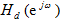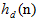是与其对应的单位脉冲响应，因此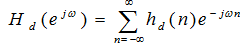（3-1）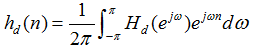（3-2)

如果我们能够在已知的情况下，求出，经过Z变换可得到滤波器的系统函数。通常情况下理想数字滤波器的单位脉冲相应是无限长的，且是非因果序列。获得有限脉冲响应滤波器的一种可能方法是对截取一段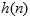来近似代替，可是这样会改变原来的滤波器指标，出现吉布斯效应误差。

窗函数法就是用被称为窗函数的有限加权序列w(n)来修正式(1)的傅里叶基数以求得要求的有限脉冲响应序列，即：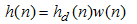（3-3）

w(n)是有限长序列，当n<0或n>N-1时，w(n)=0。

这种方法的重点在于选择某种合适的窗函数。要求窗函数主瓣宽度尽可能窄，以获得最小的过渡带；旁瓣相对值尽可能小，以使得通带波纹小，并且阻带衰减大。

下面介绍几种常用的窗函数：

1、矩形窗(Rectangle Window)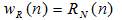（3-4）

其频率函数为：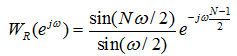（3-5）

2、三角形窗(Bartlett Window)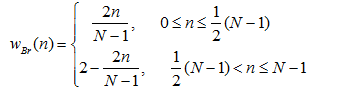（3-6）

其频率函数为：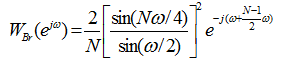（3-7)

3、汉宁(Hanning)窗，又称升余弦窗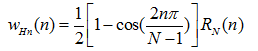（3-8）

利用傅里叶变换得到频率函数为：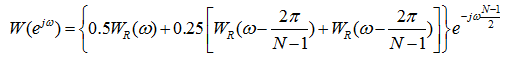（3-9）

当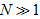时，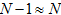，所以窗函数的幅度函数为：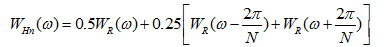(3-10)

4、汉明(Hamming)窗，又称改进的升余弦窗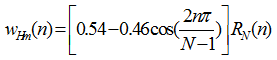（3-11）

其幅度函数为：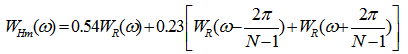（3-12）

5、布莱克曼(Blankman)窗，又称二阶升余弦窗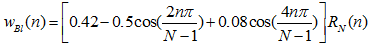（3-13)

其幅度函数为：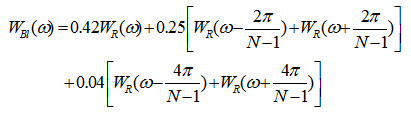(3-14）

6、凯泽(Kaiser)窗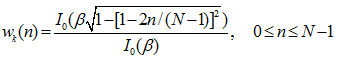（3-15）

其中：β是一个可自由选择的参数，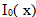是第一类修正零阶贝塞尔函数。

上述窗函数的基本参数如下表：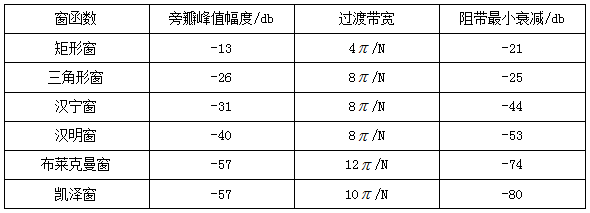窗函数法设计滤波器的步骤：

1）根据技术要求确定等待求值滤波器的单位取样响应2）根据对过渡带和阻带衰减的要求，选择窗函数的形式，并估计窗口长度N。

3）计算滤波器的单位取样响应（3-16)

上式中，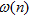是前面所选择好的窗函数。

4）检验技术指标是否满足要求。根据下式计算：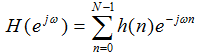(3-17)

如果不满足要求，根据具体情况重复步骤(2)(3)(4)步，直到满足要求为止。

本篇以一个FIR滤波器的设计为例说明如何使用MATLAB设计数字滤波器。

设计实例：用窗函数法设计线性相位FIR低通数字滤波器，要求通带截止频率Wp=0.4*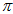，阻带截止频率Ws=0.5*, 通带衰减不大于3db，阻带衰减不小于40db。

1、程序如下：

Wp=0.4*pi;Ws=0.5*pi;Wdel=Ws-Wp;N=ceil(8*pi/Wdel);Wn=(0.4+0.5)*pi/2;window=hanning(N+1);b=fir1(N,Wn/pi,window);freqz(b,1,512)

程序执行后所得幅频和相频如下图所示：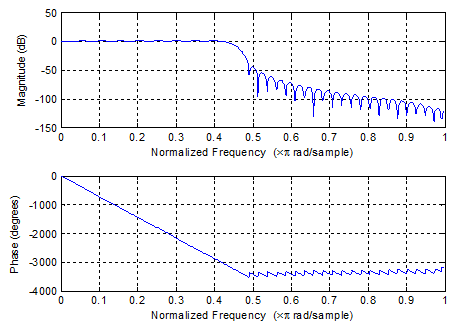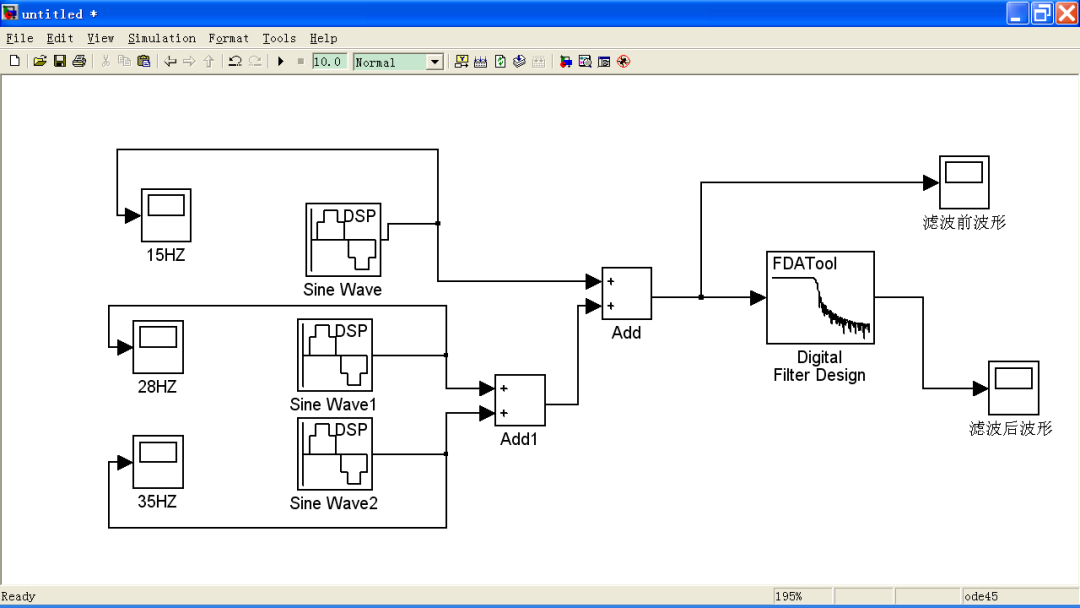输入信号：s(t)=sin(30πt)+sin(56πt)+sin(70πt)

数字滤波器参数设置：已知滤波器的阶数n=80。

首先在Filter Type中选择lowpass；

在Design Method选项中选择FIR Window，接着在Window选项中选取hann；指定Filter Order项中的Specify order为80；

采样频率Fs=100Hz,截止频率Fc=22.5HZ。

设置完以后点击窗口下方的Design Filter，在窗口上方就会看到所设计滤波器的幅频响应和相频响应曲线。

设计界面如下图所示：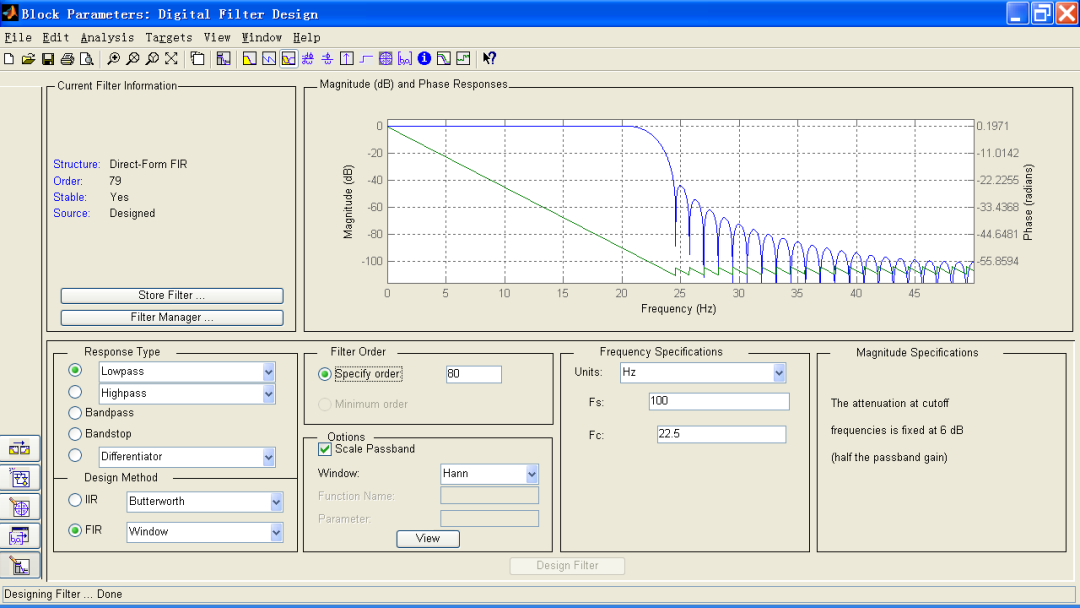信号源参数设置：三个信号源的频率分别是15Hz、28Hz、35Hz ：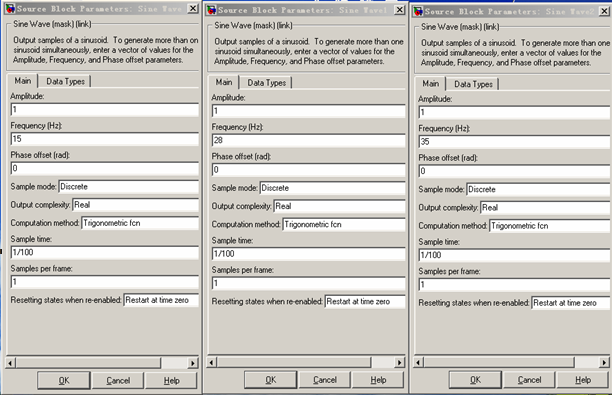运行仿真，得波形如下图所示：

15HZ信号波形如下：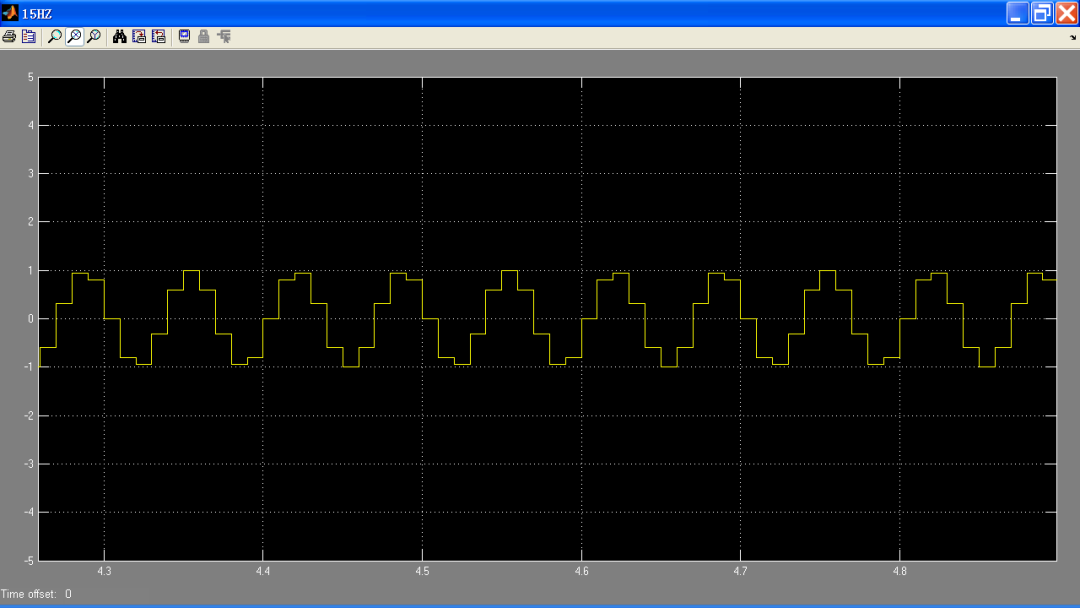28HZ信号波形如下：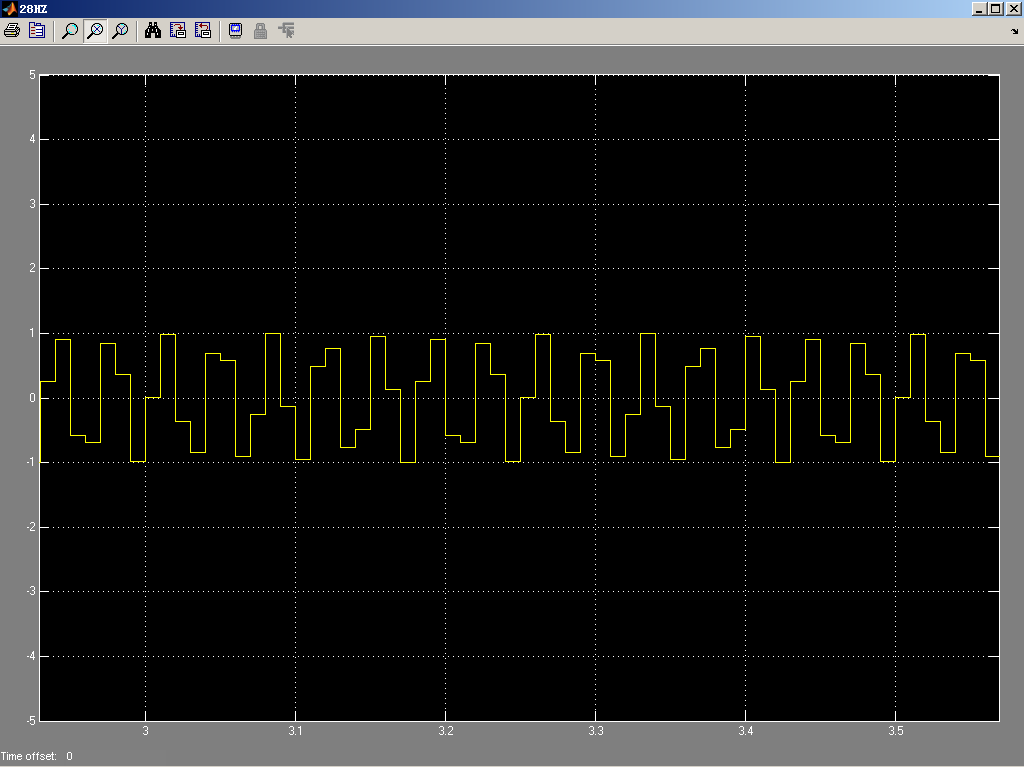35HZ信号波形如下：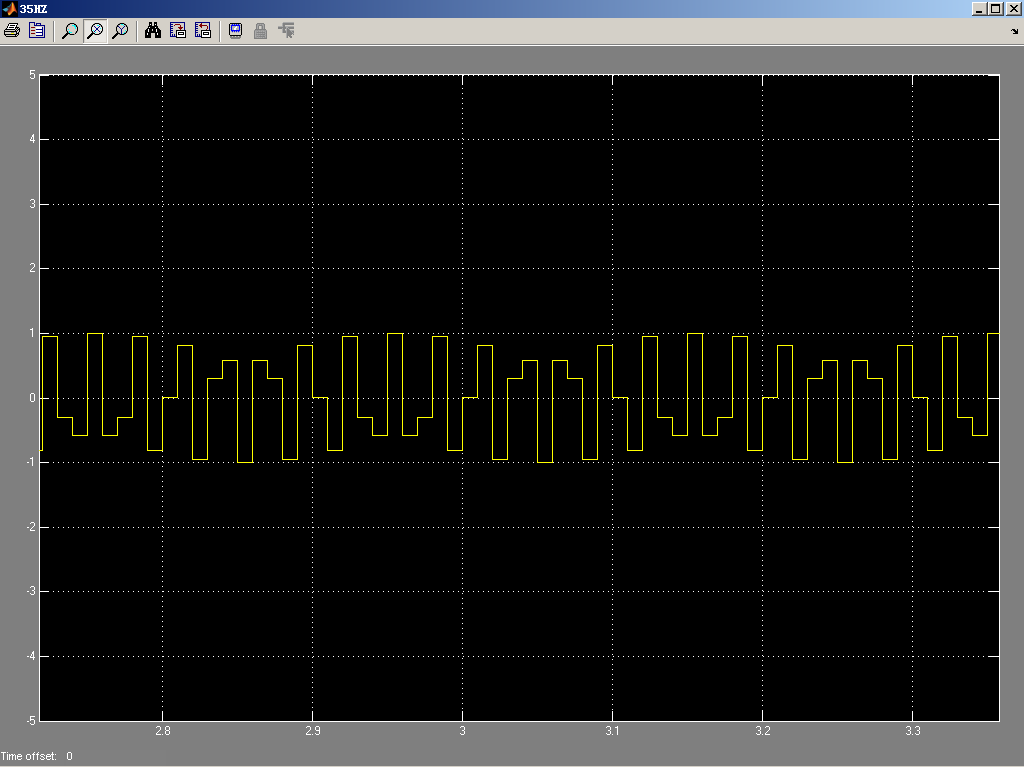滤波前的叠加波形如下：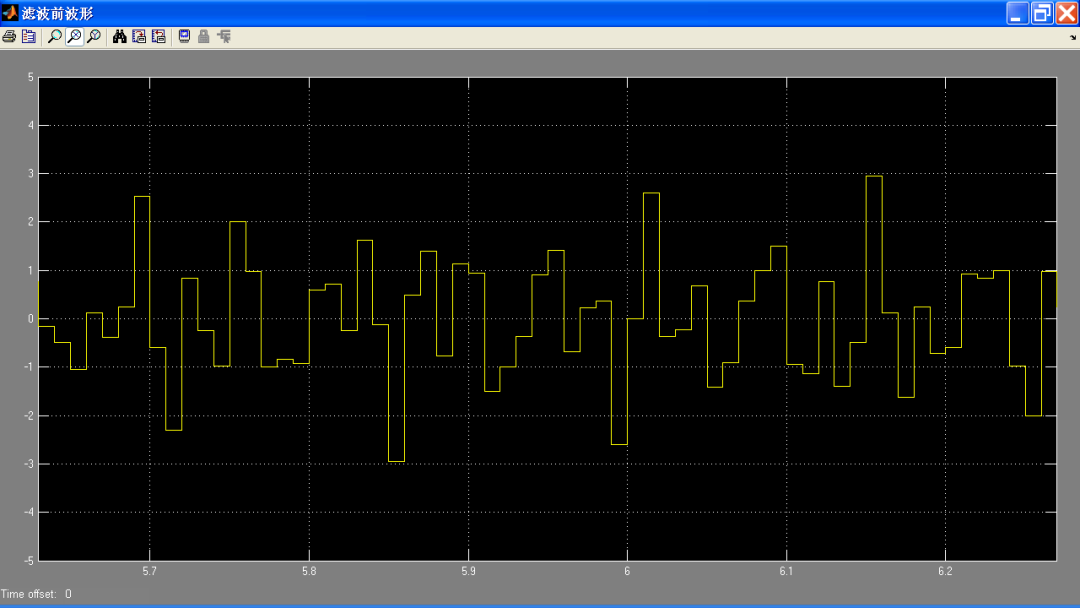滤波后波形如下：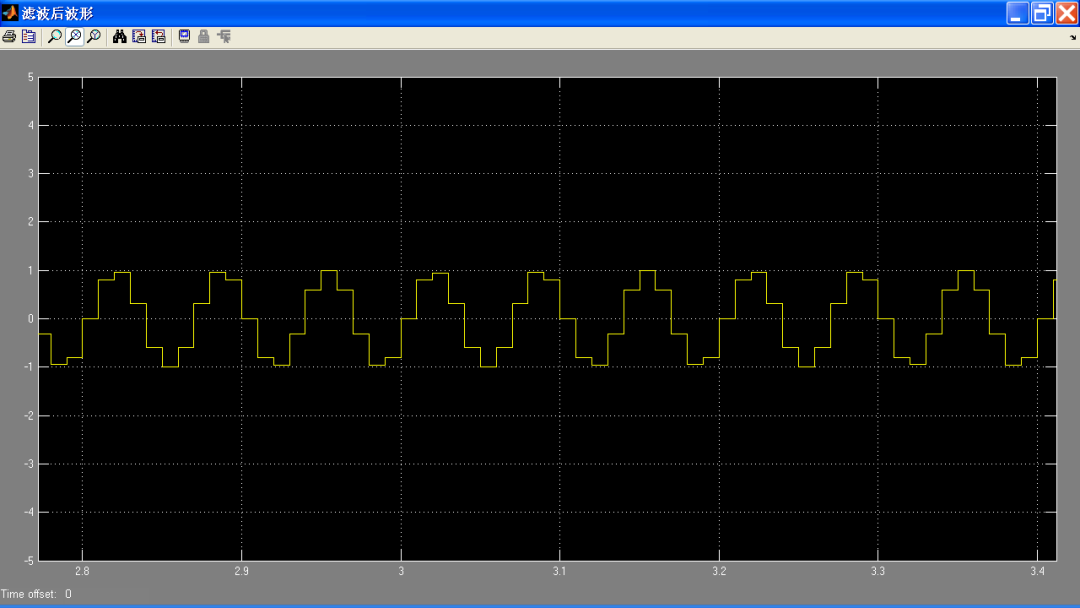由上图可知，15Hz、28Hz、35Hz叠加后通过截止频率为22.5HZ的FIR低通滤波器，输出与15Hz信号波形相似，其它的高频被阻隔。

二、频率采样法设计FIR滤波器

1、对理想滤波器的系统函数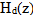进行频率采样以得到系统的理想频响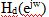的等间隔采样值H(k)。

H(k)实际上是所要求的滤波器的单位采样响应(h(n))的离散傅里叶变换（DFT），如下关系式：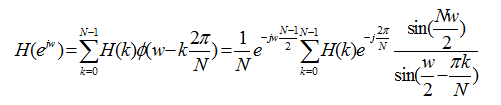(3-18)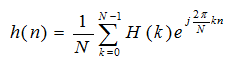(3-19)

为了减小H（k）的通带边缘由于抽样点的变化而引起的起伏振荡，可以增加过渡点，加宽过渡带以减小通带的起伏。

每一个抽样值产生一个与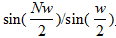成正比，并位移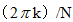的频率响应，而H（k）与内插函数的线性组合就是FIR滤波器的频率响应，增加一点过渡可以使阻带衰减提高到-44~54dB，二点过渡衰减-65~75，三点过渡衰减-85~95dB。

如果不能使过渡带太宽，同时要求增大阻带衰减，可以增加取样点数N，但这样会增加计算量、延时和误差。

频率取样型FIR滤波器设计步骤：

(1)给定理想滤波器频率响应(2)根据过渡带宽和阻带衰减确定过渡点数和h(n)的长度N。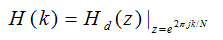(3-20)

(3)由IFFT计算IDFT得到：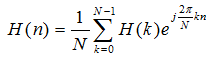(3-21)

设计实例：

频率采样法设计一个带通滤波器，

满足：低阻带边缘：w1s=0.2*；低通带边缘：w1p=0.35*；高通带边缘：w2p=0.65*；高阻带边缘：w2s=0.8*。设计过渡带中的频率样本值为t1和t2，取t1=0.109021，t2=0.59417456。

设计程序如下：

M=40;al=(M-1)/2;l=0:M-1;t1=0.109021;t2=0.59417456;Hrs=[zeros(1,5),t1,t2,ones(1,7),t2,t1,zeros(1,9),t1,t2,ones(1,7),t2,t1,zeros(1,4)];k1=0:floor((M-1)/2);k2=floor((M-1)/2)+1:M-1;angh=[-al*(2*pi)/M*k1,al*(2*pi)/M*(M-k2)];H=Hrs.*exp(j*angh);h=real(ifft(H,M));freqz(h,1,512,1000)

实验得幅频相频特性如下图所示：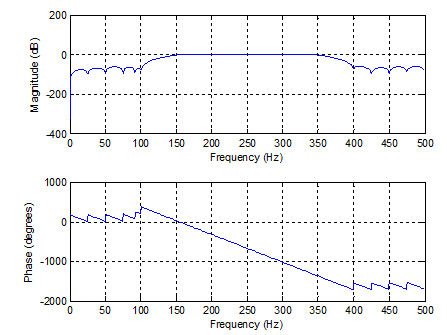三、最优化方法设计FIR数字滤波器

MATLAB信号处理工具箱提供了通用的工具函数firls和remez，采用不同的优化方法可以设计最优滤波器和多频带滤波器。

firls是fir1和fir2函数的扩展，其基本设计准则是利用最小二乘法使期望的频率响应和实际的频率响应之间的整体误差最小。

Remez函数则利用Parks-McClellan算法设计线性相位FIR滤波器。

该算法能使期望频率响应和实际频率响应之间的最大误差最小。Firls函数设计的滤波器在频率响应上出现等波纹，因此也叫等波纹滤波器。

函数firls、remez调用的语法规则相同。

1） firls。

其调用格式为：

b=firls(n,f,a)

b=firls(n,f,a,w)

b=firls(n,f,a,’ftype’)

b=firls(n,f,a,w,’ftype’)

该函数用于设计n阶FIR滤波器，其幅频特性由f和a向量确定，f是频率点向量，其范围为0~1，是递增向量；a是指定频率点的幅度响应，与f必须同长度；b为函数返回的滤波器系数，长度为n+1,且具有偶对称关系：b(k)=-b(n+2-k),  k=1,2,…,n+1；

b=firls(n,f,a,w)则使用权系数w给误差加权。

b=firls(n,f,a,w,’ftype’)调用形式中，参数’ftype’用于指定所设计的滤波器类型，ftype=Hilbert，为奇对称的线性相位滤波器。

返回的滤波器系数满足b(k)=-b(n+2-k),  k=1,2,…,n+1；ftype=differentiator，则采用特殊加权技术，生成奇对称的线性相位滤波器，使低频段误差大大小于高频段误差。

2） 函数remez的基本调用格式为：b=remez(n,f,a)。

其中各项意义同函数firls所述。

设计实例：

分别使用函数firls和remez函数设计20阶带通滤波器，阻带频率为ws1=0.3*，ws2=0.7*，通带频率为wp1=0.4*,wp2=0.6*，并画出幅频特性曲线。

1、程序如下所示：

f=[0 0.3 0.4 0.6 0.7 1];m=[0 0 1 1 0 0];n=20;b=firls(n,f,m);       %firls函数设计FIR数字滤波器[h,w]=freqz(b);     bb=remez(n,f,m);     %remez函数设计FIR数字滤波器[hh,w]=freqz(bb);axes('position',[0.2 0.2 0.5 0.5]); plot(w/pi,abs(h),'b:',w/pi,abs(hh),'r-');xlabel('frequency');ylabel('magnitude');grid on;

实验得幅频相频特性如下图所示：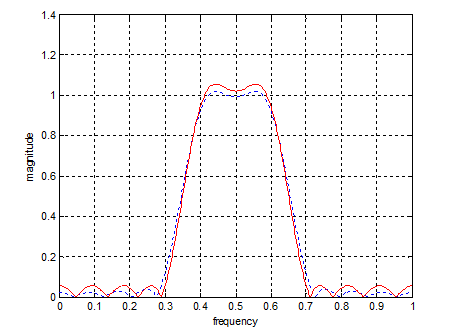仿真模块如下：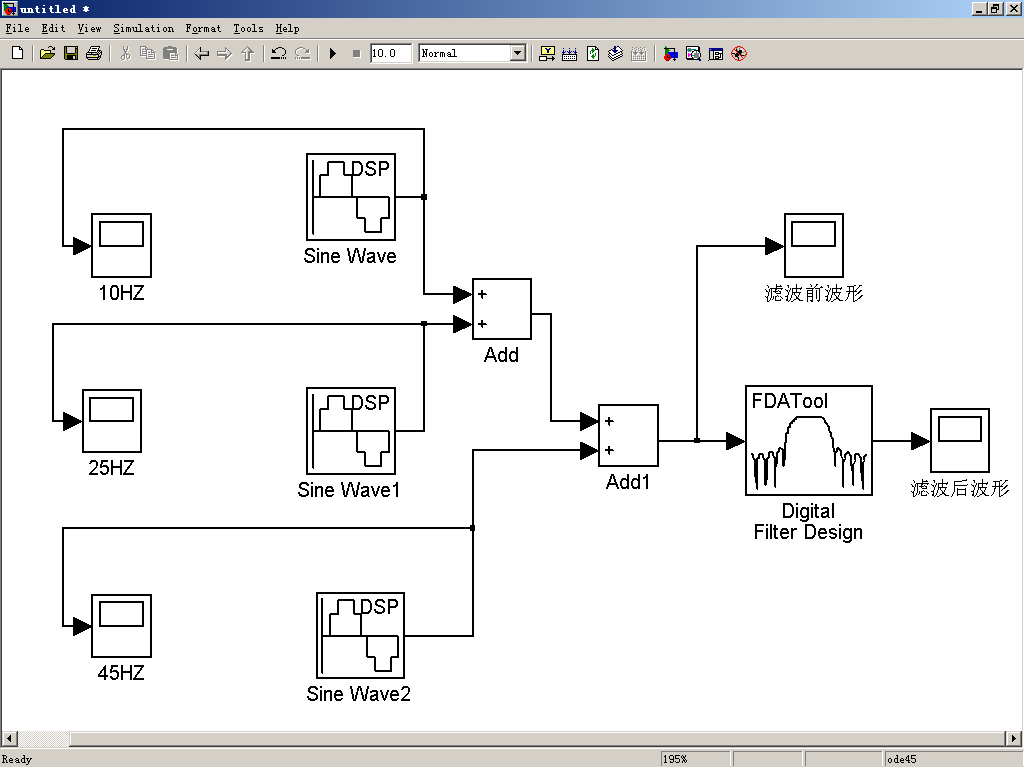输入信号：s(t)=sin(20πt)+sin(50πt)+sin(90πt)

数字滤波器参数设置：已知滤波器的阶数n=20。

首先在Filter Type中选择bandpass；

在Design Method选项中选择FIR least-squares；

指定Filter Order项中的Specify order为20；

采样频率Fs=100Hz,Fstop1=15HZ，Fpass1=20HZ，Fpass2=30HZ，Fstop2=35HZ。

设置完以后点击窗口下方的Design Filter，在窗口上方就会看到所设计滤波器的幅频响应曲线。

设计界面如下图所示：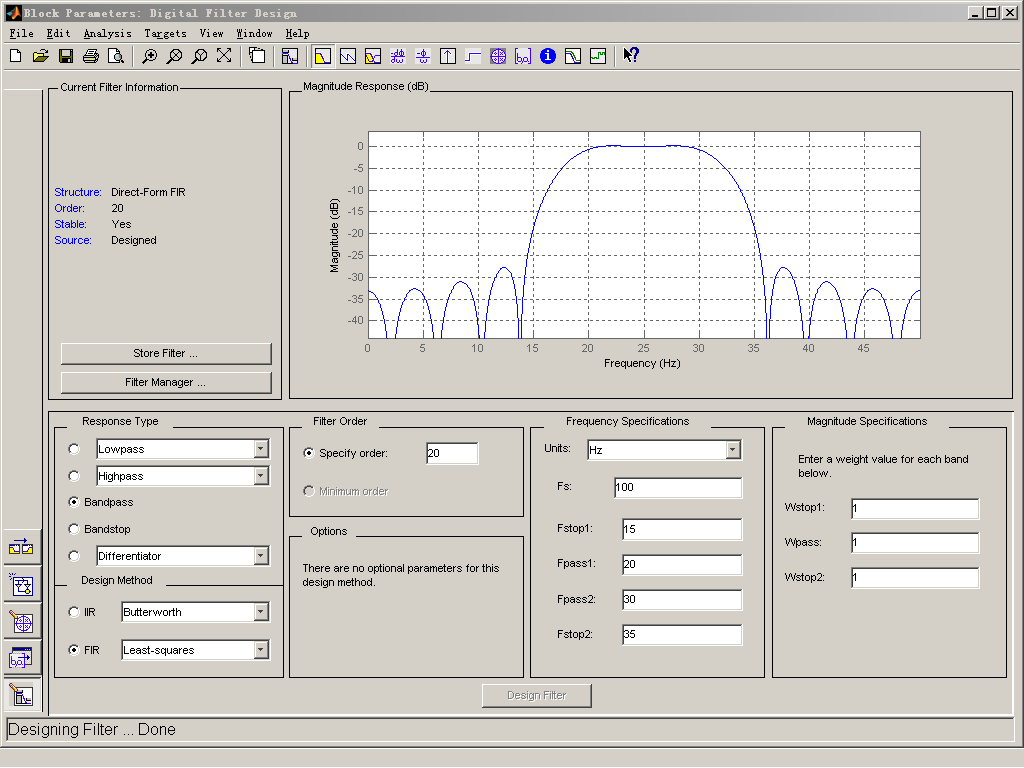信号源参数设置：三个信号源的频率分别是10Hz、25Hz、45Hz ：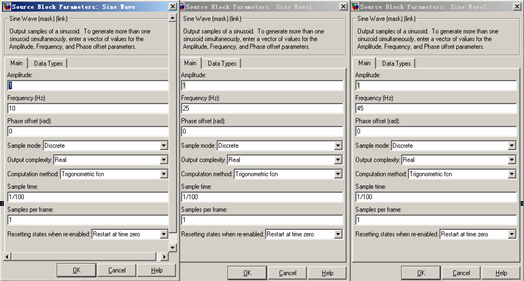运行仿真，得波形如下图所示：

（1）10HZ信号波形如下所示：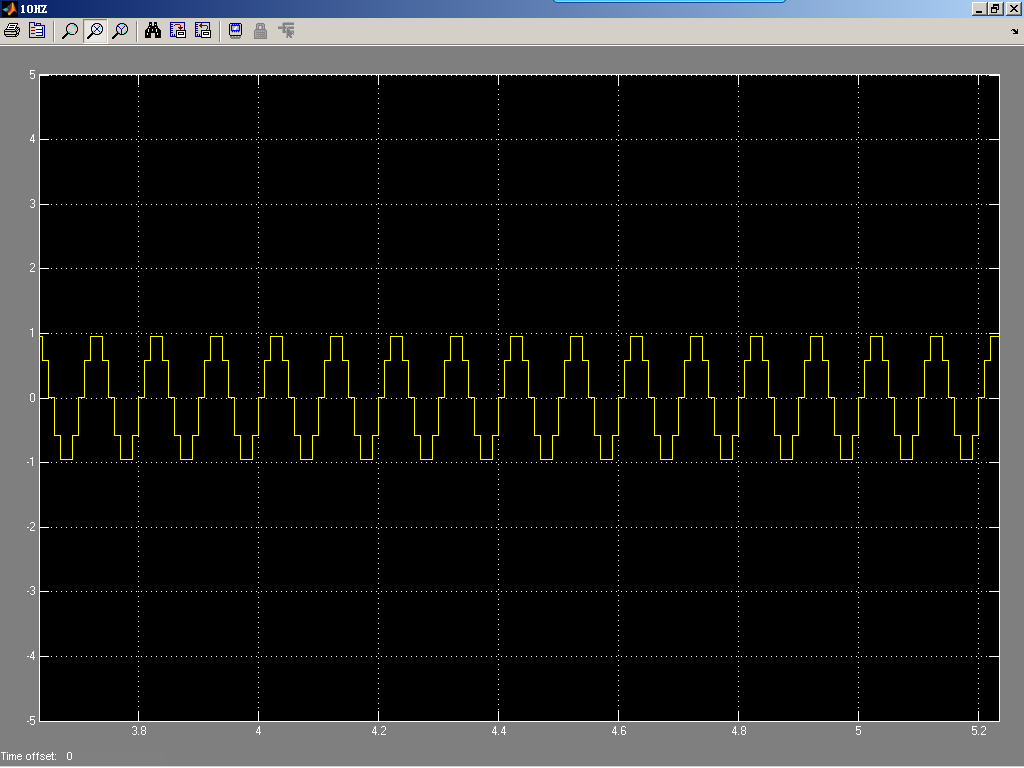（2） 25HZ信号波形如下所示：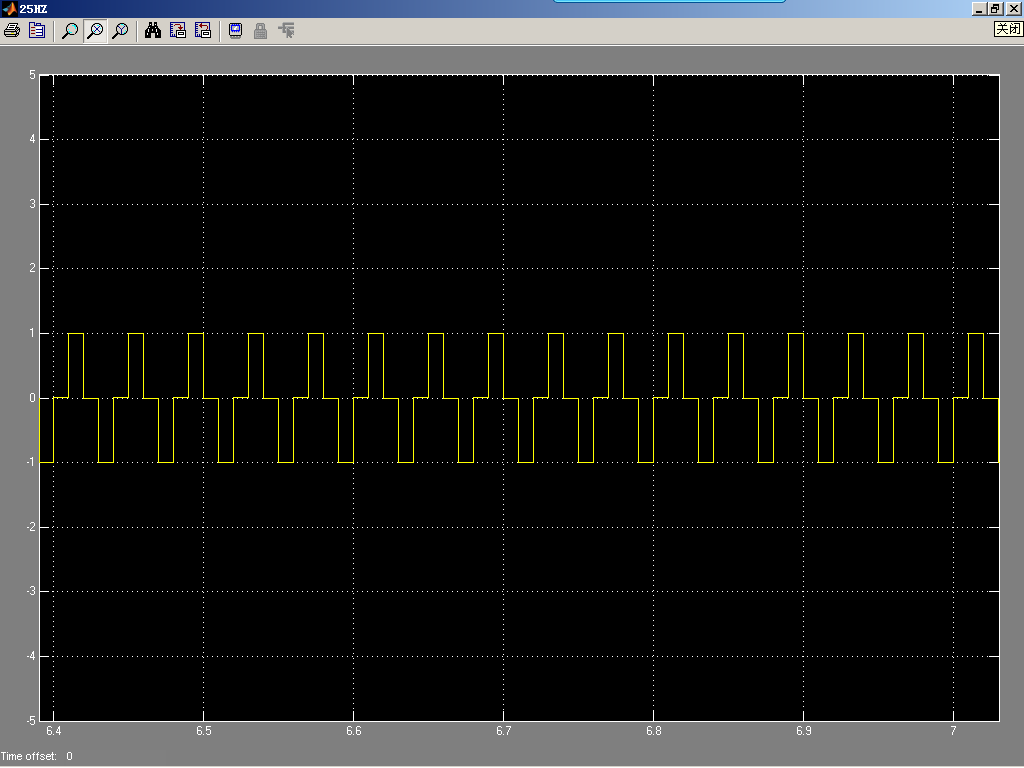（3）45HZ信号波形如下所示：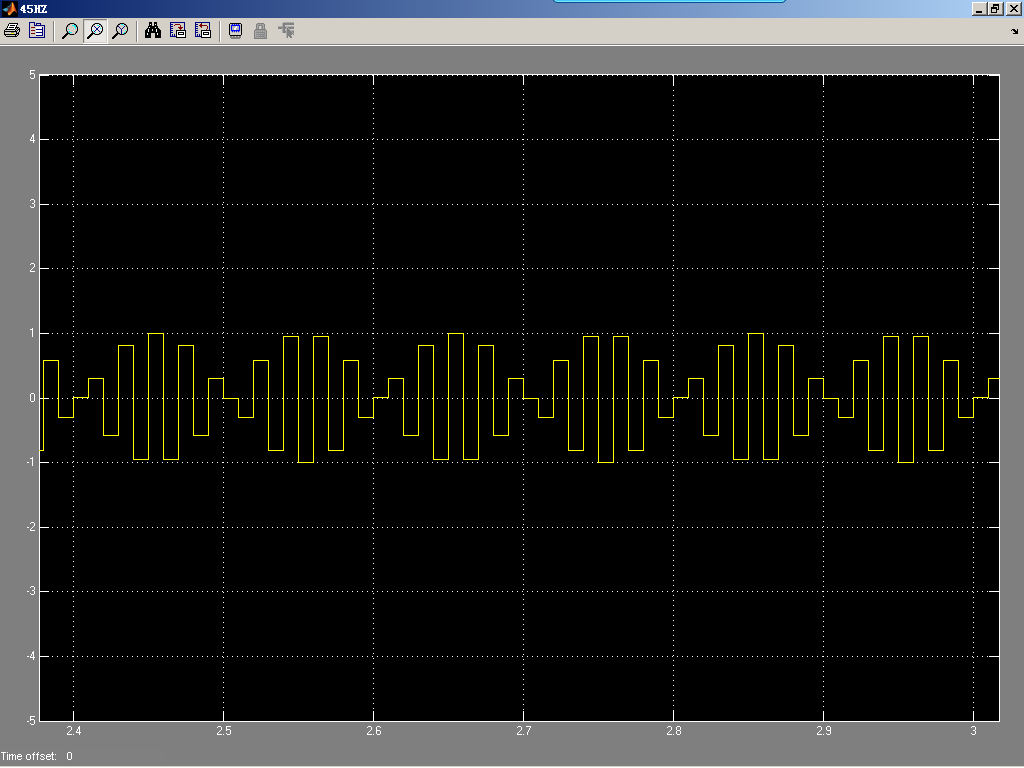（4）滤波前波形如下所示：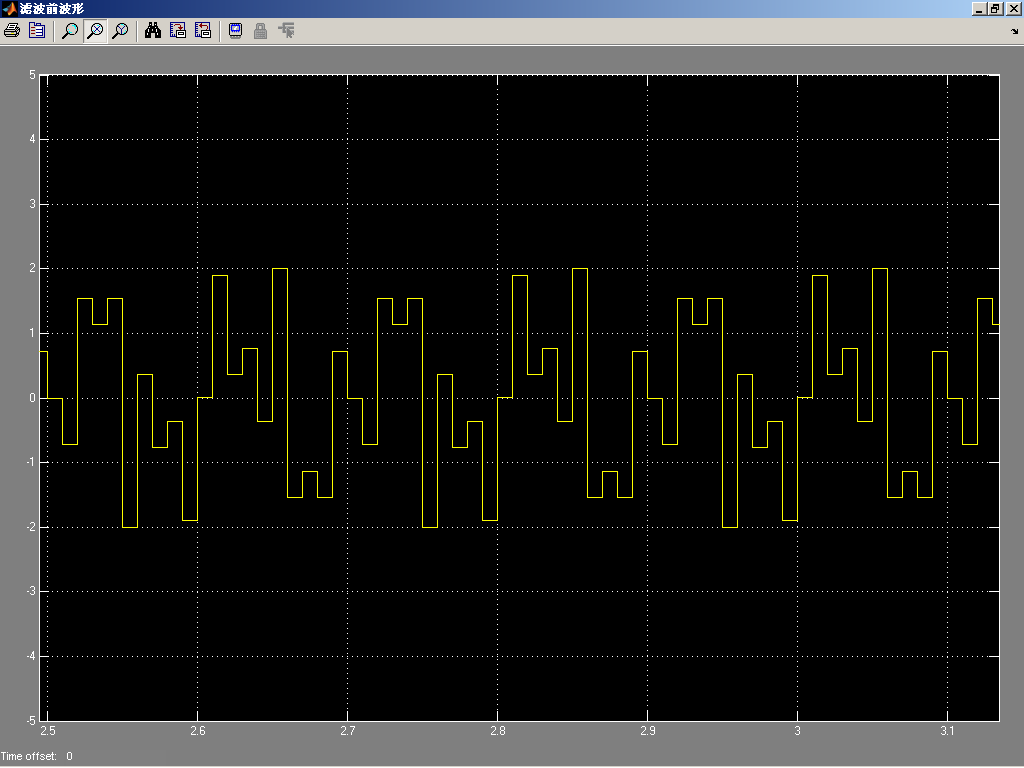（5）滤波后波形如下所示：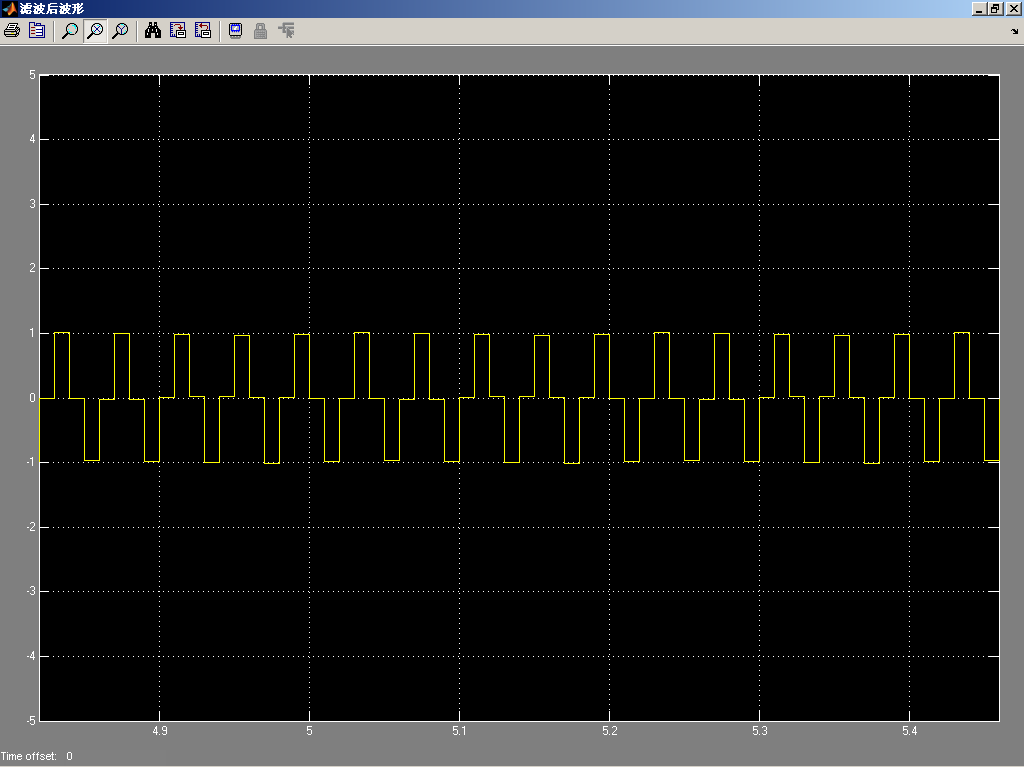由以上波形可知，10Hz、25Hz、45Hz叠加后通过通频带为20Hz~30Hz的FIR带通滤波器，输出与25Hz信号相似，低频和高频部分被阻隔。END

大侠们，江湖偌大，继续闯荡，愿一切安好，有缘再见！

往期推荐

展开全文fpga fir滤波器
• Matlab幅频曲线和滤波器设计

千次阅读 2016-09-08 15:27:50
% 求频响的幅值相位 subplot( 222 );plot(w/ pi ,magh);grid; title( 'magnitude' ); subplot( 223 );plot(w/ pi ,phah);grid; title( 'pha' ); 代码解释： zplane(B,A);其中B.A分别是H(z)的分子分母的...
• FIR:有限脉冲滤波器，线性较好，用非递归算法，可用FFT，计算速度快，不用四舍五入，适合于对相位敏感的线性应用场合,设计灵活没有现成公式可用。 　输出与现在过去的输入有关。 IIR：无限脉冲滤波器，采用递归...
• FIR滤波器实际是一个乘累加的运算，切乘累加的次数由滤波器阶数决定 结构： 串行结构：将每级延时单元与相应系数的乘积累加，因此只需要一个乘法器。系统频率是除数频率的N倍（N为乘加运算的次数） 并行结构：...
• 单位冲击响应与频响

千次阅读 2014-03-26 22:27:23
[数字信号处理]单位冲击响应与频响以及FIR实现代码（C语言） 分类： 数字信号处理 2013-05-29 13:01 993人阅读 评论(6) 收藏 举报 解卷绕FIR滤波器数字信号处理C语言 目录(?)[+] 1.单位冲击响应...
• 摘要：提出FIR敷字滤波器的设计方案，并基于Matlab实现滤波仿真。通过使用Matlab信号处理工具箱提供的函数，选择适当的窗函数编写程序，其中窗函数按照实际信号的处理需求，参数折中选择。实验获得了比较理想的...
• FIR数字滤波器的设计

万次阅读 多人点赞 2019-11-15 11:21:43
（2）熟悉线性相位FIR滤波器的幅频特性相频特性。 （3）了解各种不同窗函数对滤波器性能的影响。 二、实验内容 (1)N=45，计算并画出矩形窗、汉明窗、布莱克曼窗的归一化的幅度谱， 并比较各自的主要特点。 矩形窗:...filter
• fir数字滤波器设计及matlab使用.doc 数字信号处理课程设计数字信号处理课程设计报告FIR数字滤波器设计及MATLAB实现专业通信工程班级通信1101班组次第9组姓名及学号姓名及学号数字信号处理课程设计第1页组员承担任务...
• (二)FIR数字滤波器的FPGA实现-串行FIR滤波器设计 ...  对于FIR滤波器主要涉及到滤波器的设计滤波器的实现，设计实现的区别如下图所示：   实现是 forward problem，设计是 inverse prob...
• FIR滤波器设计

千次阅读 2020-12-14 15:10:32
1、H = dsp.FIRFilter返回默认的FIR过滤器对象H，该对象使用指定的FIR过滤器实现在对step方法的连续调用中，独立过滤输入的每个通道。 2、H = dsp.FIRFilter（'PropertyName'，PropertyValue，...）返回FIR
• 低通fp=1000;fc=1200;As=100;Ap=1;Fs=3000;wc=2*pi*fc/Fs; wp=2*pi*fp/Fs;wd=wc-wp;beta=0。1102*(As-8。7);N=ceil((As-7。95)/2。286/wd);wn= kaiser(N 1,beta);...b=fir1(N,ws,wn);figure(1);freqz(b,1);x=...
• 实验4 基于MATLAB 的FIR 数字滤波器设计一、 实验目的(1) 加深对数字滤波器的常用指标理解。 (2) 学习数字滤波器的设计方法。 二、 实验原理 低通滤波器1()1()P P P SS H H δδδπ?-≤Ω≤+Ω≤Ω??Ω≤Ω≤Ω≤??...
• 实验四参考基于matlab的fir数字滤波器设计 实验 4 基于 MATLAB 的 FIR 数字滤波器设计一、 实验目的(1) 加深对数字滤波器的常用指标理解。(2) 学习数字滤波器的设计方法。二、 实验原理低通滤波器 1()1PPPSSH...
• 实验五：FIR 数字滤波器设计与软件实现一、实验目的(1)掌握用窗函数法设计FIR 数字滤波器的原理方法。(2)掌握用等波纹最佳逼近法设计FIR 数字滤波器的原理方法。(3)掌握FIR 滤波器的快速卷积实现原理。(4)学会...
• 毕设：基于FPGA的FIR数字滤波器设计

千次阅读 多人点赞 2020-06-28 10:07:14
（一）掌握有限冲击响应FIR（Finite Impulse Response， FIR）的基本结构，研究现有的实现方法，对各种方案步骤进行比较论证分析，然后针对目前FIR数字滤波器需要的特点，速度快硬件规模小，作为指导思想进行...fpga FIR滤波器
• 实验目的(1)掌握用窗函数法设计FIR数字滤波器的原理方法。(2)掌握用等波纹最佳逼近法设计FIR数字滤波器的原理方法。(3)掌握FIR滤波器的快速卷积实现原理。(4)学会调用MATLAB函数设计与实现FIR滤波器。2． 实验...
• 使用工具 MATLAB R2014b、Quartus ...在FIR的脚本仿真文章中已介绍过，设计一个10M采样率，200KHz截止频率的FIR低通滤波器，将fir_lpf_200K_10M.txt文件复制到FIR IP核目录中。 第二步 新建Quartus工程，生成IP核 1）QuFPGA ModelSim
• 目录：MATLAB-FIR数字滤波器设计1 FIR滤波器的原理2 FIR滤波器的特点2.1 相位特性2.1.1 偶对称2.1.2 奇对称2.2 幅度特性3 几种滤波器函数3.1 fir1()3.2 fir2()3.3 kaiserord()3.4 firpm()4 filterDesigner配置4.1 ...matlab 数字信号处理 数字通信
• 实验6 基于MATLAB 的FIR 数字滤波器设计一、 实验目的(1) 加深对数字滤波器的常用指标理解。 (2) 学习数字滤波器的设计方法。 二、 实验原理 低通滤波器1()1()P P P SS H H δδδπ?-≤Ω≤+Ω≤Ω??Ω≤Ω≤Ω≤??...
• 窗函数法设计FIRDF使用Matlab的fir1（）函数十分方便，DSP课堂上已经讲过，此处不再赘述。关心的是：使用 hn = fir1（M， wc）；%M为滤波器阶数M，长度N， M = N-1,；wc为截止频率得到向量hn之后，如何用freqz表示？...数字信号处理 滤波器设计 Matlab
• matlab已知滤波器参数,求频响

千次阅读 2018-07-16 13:59:57
例：y(n)=x(n)-x(n-3) %减法滤波器 clear all; clc; fs=600; b=[1,0,0,-1]; a=; [h,f]=freqz(b,a,256,fs); mag=abs(h); ph=angle(h); ph=ph*180/pi; subplot(2,1,1) ...xlabel(‘频率(Hz)’...
• 1、熟悉线性相位FIR滤波器的幅频特性相频特性； 2、加深对窗函数法设计FIR数字滤波器的基本原理的理解； 3、了解Matlab有关窗函数法设计的子函数以及各种不同窗函数对滤波器性能的影响。 实验原理： 根据上式中的...
• FIR滤波器设计通常可以分为窗函数法频率采样法两类，这里先介绍窗函数法 FIR 滤波器简介 考虑一个N−1N−1阶FIR滤波器 z变换z变换： H(z)=∑N−1n=0h(n)z−n H(z)= n=0 ∑ N−1 ​ h(n)z −n 差分方程： y(n)=...python
• 窗函数法设计FIR滤波器与IIR滤波器Python编写(从MATLAB移植） 1. 题目要求 2. MATLAB代码 来自Peter_831 clc; clear; close all; Fs=4000; % 采样频率4000Hz t=0:1/Fs:1; s50=sin(2*pi*50*t); % 产生50Hz正弦波 ......